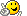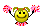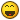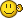Jump to content
• Sign Up

## Recommended Posts

I've been working on a calculator function for another addition for my "simple" collection of tiny maybe useless apps. I'm having trouble with the reset function on the calculator:

```#include <GUICONSTANTS.au3>

_Calculator()

Func _Calculator()
Global \$Multiply = 0, \$Divide = 0, \$Addition = 0, \$Subtraction = 0
\$Calculator = GUICreate("Simple Calculator", 223, 130, 193, 115)
\$Input1 = GUICtrlCreateInput("", 0, 0, 121, 21)
\$1 = GUICtrlCreateButton("1", 0, 32, 19, 25, 0)
\$2 = GUICtrlCreateButton("2", 24, 32, 19, 25, 0)
\$3 = GUICtrlCreateButton("3", 48, 32, 19, 25, 0)
\$4 = GUICtrlCreateButton("4", 72, 32, 19, 25, 0)
\$5 = GUICtrlCreateButton("5", 96, 32, 19, 25, 0)
\$6 = GUICtrlCreateButton("6", 0, 64, 19, 25, 0)
\$7 = GUICtrlCreateButton("7", 24, 64, 19, 25, 0)
\$8 = GUICtrlCreateButton("8", 48, 64, 19, 25, 0)
\$9 = GUICtrlCreateButton("9", 72, 64, 19, 25, 0)
\$0 = GUICtrlCreateButton("0", 96, 64, 19, 25, 0)
\$Multiply = GUICtrlCreateButton("*", 0, 104, 19, 25, 0)
\$Divide = GUICtrlCreateButton("/", 24, 104, 19, 25, 0)
\$Addition = GUICtrlCreateButton("+", 48, 104, 19, 25, 0)
\$Subtraction = GUICtrlCreateButton("-", 72, 104, 19, 25, 0)
\$Equals = GUICtrlCreateButton("=", 96, 104, 19, 25, 0)
\$Reset = GUICtrlCreateButton("Reset", 120, 104)
GUISetState(@SW_SHOW)
While 1
\$CalculatorMsg = GUIGetMsg()
Switch \$CalculatorMsg
Case \$GUI_EVENT_CLOSE
GUIDelete(\$Calculator)
ExitLoop
Case \$1
\$ReadCurrentOutput = GUICtrlRead(\$Input1)
GUICtrlSetData(\$Input1, \$ReadCurrentOutput & "1")
Case \$2
\$ReadCurrentOutput = GUICtrlRead(\$Input1)
GUICtrlSetData(\$Input1, \$ReadCurrentOutput & "2")
Case \$3
\$ReadCurrentOutput = GUICtrlRead(\$Input1)
GUICtrlSetData(\$Input1, \$ReadCurrentOutput & "3")
Case \$4
\$ReadCurrentOutput = GUICtrlRead(\$Input1)
GUICtrlSetData(\$Input1, \$ReadCurrentOutput & "4")
Case \$5
\$ReadCurrentOutput = GUICtrlRead(\$Input1)
GUICtrlSetData(\$Input1, \$ReadCurrentOutput & "5")
Case \$6
\$ReadCurrentOutput = GUICtrlRead(\$Input1)
GUICtrlSetData(\$Input1, \$ReadCurrentOutput & "6")
Case \$7
\$ReadCurrentOutput = GUICtrlRead(\$Input1)
GUICtrlSetData(\$Input1, \$ReadCurrentOutput & "7")
Case \$8
\$ReadCurrentOutput = GUICtrlRead(\$Input1)
GUICtrlSetData(\$Input1, \$ReadCurrentOutput & "8")
Case \$9
\$ReadCurrentOutput = GUICtrlRead(\$Input1)
GUICtrlSetData(\$Input1, \$ReadCurrentOutput & "9")
Case \$0
\$ReadCurrentOutput = GUICtrlRead(\$Input1)
GUICtrlSetData(\$Input1, \$ReadCurrentOutput & "0")
Case \$Multiply
\$Multiply = 1
\$MultiplyReadBefore = GUICtrlRead(\$Input1)
GUICtrlSetData(\$Input1, "")
Case \$Divide
\$Divide = 1
\$DivideReadBefore = GUICtrlRead(\$Input1)
GUICtrlSetData(\$Input1, "")
Case \$Addition
\$Addition = 1
\$AdditionReadBefore = GUICtrlRead(\$Input1)
GUICtrlSetData(\$Input1, "")
Case \$Subtraction
\$Subtraction = 1
\$SubtractionReadBefore = GUICtrlRead(\$Input1)
GUICtrlSetData(\$Input1, "")
Case \$Equals
\$ReadAllAfter = GUICtrlRead(\$Input1)
If \$Multiply = 1 Then
\$Answer = Number(\$MultiplyReadBefore * \$ReadAllAfter)
GUICtrlSetData(\$Input1, \$Answer)
EndIf
If \$Divide = 1 Then
\$Answer = Number(\$DivideReadBefore / \$ReadAllAfter)
GUICtrlSetData(\$Input1, \$Answer)
EndIf
If \$Addition = 1 Then
\$Answer = Number(\$AdditionReadBefore + \$ReadAllAfter)
GUICtrlSetData(\$Input1, \$Answer)
EndIf
If \$Subtraction = 1 Then
\$Answer = Number(\$SubtractionReadBefore - \$ReadAllAfter)
GUICtrlSetData(\$Input1, \$Answer)
EndIf
Case \$Reset
\$Multiply = 0
\$Divide = 0
\$Addition = 0
\$Subtraction = 0
GuiCtrlSetData(\$Input1, "")
EndSwitch
WEnd
EndFunc   ;==>_Calculator```

It doesn't work after you get your answer. Anyone know why? I've tried Global variables, removing them, all this crazy stuff, but any help is highly appreciated! Thanks!#### Share this post

##### Share on other sites

Simple:

```Case \$Reset
\$Multiply = 0
\$Divide = 0
\$Addition = 0
\$Subtraction = 0```

If you look your script you'll see that \$Multiply, \$Divide, etc are controlID's!!

#### Share this post

##### Share on other sites

Ahh, I get it. Thanks!

#### Share this post

##### Share on other sites

Here.

```#include <GUICONSTANTS.au3>

_Calculator()

Func _Calculator()
Global \$Multiply = 0, \$Divide = 0, \$Addition = 0, \$Subtraction = 0
\$Calculator = GUICreate("Simple Calculator", 223, 130, 193, 115)
\$Input1 = GUICtrlCreateInput("", 0, 0, 121, 21)
\$1 = GUICtrlCreateButton("1", 0, 32, 19, 25, 0)
\$2 = GUICtrlCreateButton("2", 24, 32, 19, 25, 0)
\$3 = GUICtrlCreateButton("3", 48, 32, 19, 25, 0)
\$4 = GUICtrlCreateButton("4", 72, 32, 19, 25, 0)
\$5 = GUICtrlCreateButton("5", 96, 32, 19, 25, 0)
\$6 = GUICtrlCreateButton("6", 0, 64, 19, 25, 0)
\$7 = GUICtrlCreateButton("7", 24, 64, 19, 25, 0)
\$8 = GUICtrlCreateButton("8", 48, 64, 19, 25, 0)
\$9 = GUICtrlCreateButton("9", 72, 64, 19, 25, 0)
\$0 = GUICtrlCreateButton("0", 96, 64, 19, 25, 0)
\$Mult = GUICtrlCreateButton("*", 0, 104, 19, 25, 0)
\$Div = GUICtrlCreateButton("/", 24, 104, 19, 25, 0)
\$Add = GUICtrlCreateButton("+", 48, 104, 19, 25, 0)
\$Sub = GUICtrlCreateButton("-", 72, 104, 19, 25, 0)
\$Equ = GUICtrlCreateButton("=", 96, 104, 19, 25, 0)
\$Reset = GUICtrlCreateButton("Reset", 120, 104)
GUISetState(@SW_SHOW)
While 1
\$CalculatorMsg = GUIGetMsg()
Switch \$CalculatorMsg
Case \$GUI_EVENT_CLOSE
GUIDelete(\$Calculator)
ExitLoop
Case \$1
\$ReadCurrentOutput = GUICtrlRead(\$Input1)
GUICtrlSetData(\$Input1, \$ReadCurrentOutput & "1")
Case \$2
\$ReadCurrentOutput = GUICtrlRead(\$Input1)
GUICtrlSetData(\$Input1, \$ReadCurrentOutput & "2")
Case \$3
\$ReadCurrentOutput = GUICtrlRead(\$Input1)
GUICtrlSetData(\$Input1, \$ReadCurrentOutput & "3")
Case \$4
\$ReadCurrentOutput = GUICtrlRead(\$Input1)
GUICtrlSetData(\$Input1, \$ReadCurrentOutput & "4")
Case \$5
\$ReadCurrentOutput = GUICtrlRead(\$Input1)
GUICtrlSetData(\$Input1, \$ReadCurrentOutput & "5")
Case \$6
\$ReadCurrentOutput = GUICtrlRead(\$Input1)
GUICtrlSetData(\$Input1, \$ReadCurrentOutput & "6")
Case \$7
\$ReadCurrentOutput = GUICtrlRead(\$Input1)
GUICtrlSetData(\$Input1, \$ReadCurrentOutput & "7")
Case \$8
\$ReadCurrentOutput = GUICtrlRead(\$Input1)
GUICtrlSetData(\$Input1, \$ReadCurrentOutput & "8")
Case \$9
\$ReadCurrentOutput = GUICtrlRead(\$Input1)
GUICtrlSetData(\$Input1, \$ReadCurrentOutput & "9")
Case \$0
\$ReadCurrentOutput = GUICtrlRead(\$Input1)
GUICtrlSetData(\$Input1, \$ReadCurrentOutput & "0")
Case \$Mult
\$Multiply = 1
\$MultiplyReadBefore = GUICtrlRead(\$Input1)
GUICtrlSetData(\$Input1, "")
Case \$Div
\$Divide = 1
\$DivideReadBefore = GUICtrlRead(\$Input1)
GUICtrlSetData(\$Input1, "")
Case \$Add
\$Addition = 1
\$AdditionReadBefore = GUICtrlRead(\$Input1)
GUICtrlSetData(\$Input1, "")
Case \$Sub
\$Subtraction = 1
\$SubtractionReadBefore = GUICtrlRead(\$Input1)
GUICtrlSetData(\$Input1, "")
Case \$Equ
\$ReadAllAfter = GUICtrlRead(\$Input1)
If \$Multiply = 1 Then
\$Answer = Number(\$MultiplyReadBefore * \$ReadAllAfter)
GUICtrlSetData(\$Input1, \$Answer)
EndIf
If \$Divide = 1 Then
\$Answer = Number(\$DivideReadBefore / \$ReadAllAfter)
GUICtrlSetData(\$Input1, \$Answer)
EndIf
If \$Addition = 1 Then
\$Answer = Number(\$AdditionReadBefore + \$ReadAllAfter)
GUICtrlSetData(\$Input1, \$Answer)
EndIf
If \$Subtraction = 1 Then
\$Answer = Number(\$SubtractionReadBefore - \$ReadAllAfter)
GUICtrlSetData(\$Input1, \$Answer)
EndIf
Case \$Reset
\$Multiply = 0
\$Divide = 0
\$Addition = 0
\$Subtraction = 0
GuiCtrlSetData(\$Input1, "")
EndSwitch
WEnd
EndFunc   ;==>_Calculator```

#### Share this post

##### Share on other sites

Ok, I fixed it, I ws thinking using the control also as a variable with the same name!Thanks!!:P

EDIT: I didn't see your script, I fixed it myself, thanks though!Edited by JustinReno

## Create an account or sign in to comment

You need to be a member in order to leave a comment

## Create an account

Sign up for a new account in our community. It's easy!

Register a new account

## Sign in

Already have an account? Sign in here.

Sign In Now

• ### Recently Browsing   0 members

No registered users viewing this page.

×

• Wiki

• Back

• #### Beta

• Git
• FAQ
• Our Picks
×
• Create New...